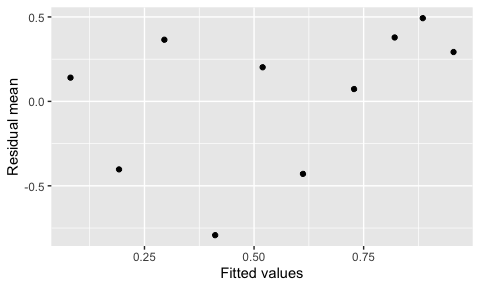# Logistic regression diagnostics

library(regressinator)
library(dplyr)
library(ggplot2)
library(broom)

For these examples of logistic regression diagnostics, we’ll consider a simple bivariate setting where the model is misspecified:

logistic_pop <- population(
x1 = predictor("rnorm", mean = 0, sd = 10),
x2 = predictor("runif", min = 0, max = 10),
y = response(0.7 + 0.2 * x1 + x1^2 / 100 - 0.2 * x2,
)

logistic_data <- sample_x(logistic_pop, n = 100) |>
sample_y()

fit <- glm(y ~ x1 + x2, data = logistic_data, family = binomial)

In other words, the population relationship is \begin{align*} Y \mid X = x &\sim \text{Bernoulli}(\mu(x)) \\ \mu(x) &= \operatorname{logit}^{-1}\left(0.7 + 0.2 x_1 + \frac{x_1^2}{100} - 0.2 x_2\right), \end{align*} but we chose to fit a model that does not allow a quadratic term for $$x_1$$.

## Empirical logit plots

Before fitting the model, we might conduct exploratory data analysis to determine what model is appropriate. In linear regression, scatterplots of the predictors versus the response variable would be helpful, but with a binary outcome these are much harder to interpret.

Instead, an empirical logit plot can help us visualize the relationship between predictor and response. We break the range of x1 into bins, and within each bin, calculate the mean value of x1 and y for observations in that bin. We then transform the mean of y through the link function; in logistic regression, this is the logit, so we transform from a fraction to the log-odds. If the logistic model is well-specified, x1 and the logit of y should be linearly related. The logits of 0 and 1 are $$-\infty$$ and $$+\infty$$, so taking averages of y within bins ensures the logits are on a more reasonable range.

The bin_by_quantile() function groups the data into bins, while empirical_link() can calculate the empirical value of a variable on the link scale, for any GLM family:

logistic_data |>
bin_by_quantile(x1, breaks = 6) |>
summarize(x = mean(x1),
ggplot(aes(x = x, y = response)) +
geom_point() +
labs(x = "X1", y = "logit(Y)")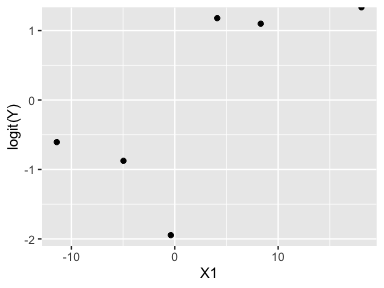This looks suspiciously nonlinear.

Similarly for x2:

logistic_data |>
bin_by_quantile(x2, breaks = 6) |>
summarize(x = mean(x2),
ggplot(aes(x = x, y = response)) +
geom_point() +
labs(x = "X2", y = "logit(Y)")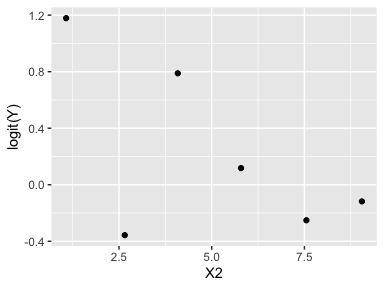This looks more linear, though it is difficult to assess. We could also use model_lineup() to examine similar plots when the model is correctly specified, to tell if these plots indicate a serious problem.

## Naive residual plots

Once we have fit the model, ordinary standardized residuals are not very helpful for noticing the misspecification:

augment(fit) |>
ggplot(aes(x = .fitted, y = .std.resid)) +
geom_point() +
geom_smooth(se = FALSE) +
labs(x = "Fitted value", y = "Residual")
#> geom_smooth() using method = 'loess' and formula = 'y ~ x'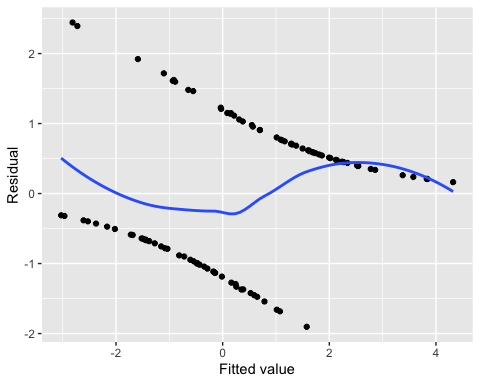Nor are plots of standardized residuals against the predictors:

augment_longer(fit) |>
ggplot(aes(x = .predictor_value, y = .std.resid)) +
geom_point() +
geom_smooth(se = FALSE) +
facet_wrap(vars(.predictor_name), scales = "free_x") +
labs(x = "Predictor", y = "Residual")
#> geom_smooth() using method = 'loess' and formula = 'y ~ x'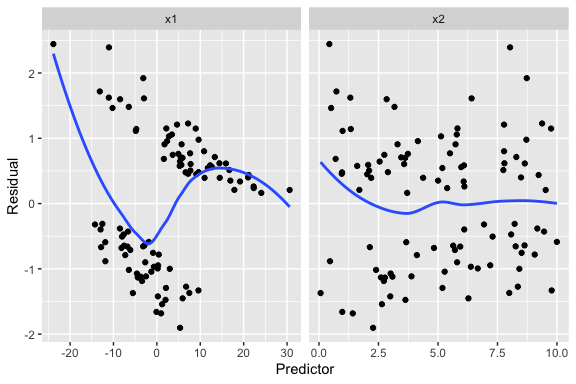We see a hint of something in the smoothed line on the left, but it is hard to judge what that means. Because our outcome is binary, the residuals are divided into two clumps ($$Y = 0$$ and $$Y = 1$$), making their distribution hard to interpret and trends hard to spot.

## Marginal model plots

For each predictor, we plot the predictor versus $$Y$$. We plot the smoothed curve of fitted values (red) as well as a smoothed curve of response values (blue):

augment_longer(fit, type.predict = "response") |>
ggplot(aes(x = .predictor_value)) +
geom_point(aes(y = y)) +
geom_smooth(aes(y = .fitted), color = "red") +
geom_smooth(aes(y = y)) +
facet_wrap(vars(.predictor_name), scales = "free_x") +
labs(x = "Predictor", y = "Y")
#> geom_smooth() using method = 'loess' and formula = 'y ~ x'
#> geom_smooth() using method = 'loess' and formula = 'y ~ x'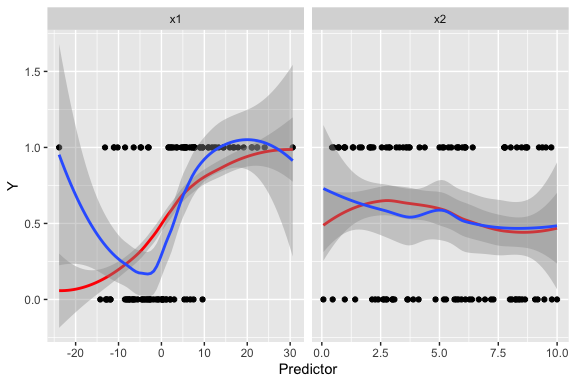The red line is a smoothed version of $$\hat \mu(x)$$ versus $$X_1$$, while the blue line averages $$Y$$ (which is 0 or 1, so the average is the true fraction of 1s) versus $$X_1$$. Comparing the two lines helps us evaluate if the model is well-specified.

This again suggests something may be going on with x1, but it’s hard to tell what specifically might be wrong.

## Partial residuals

The partial residuals make the quadratic shape of the relationship much clearer:

partial_residuals(fit) |>
ggplot(aes(x = .predictor_value, y = .partial_resid)) +
geom_point() +
geom_smooth() +
geom_line(aes(x = .predictor_value, y = .predictor_effect)) +
facet_wrap(vars(.predictor_name), scales = "free") +
labs(x = "Predictor", y = "Partial residual")
#> geom_smooth() using method = 'loess' and formula = 'y ~ x'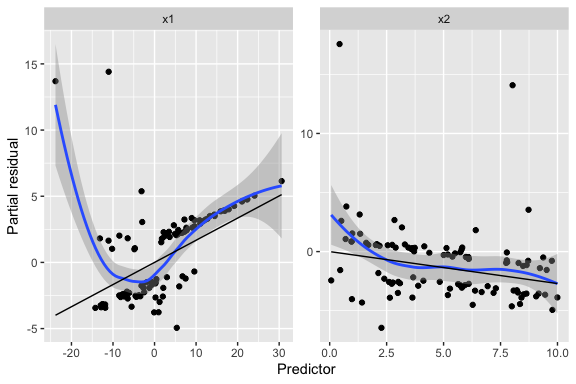See the partial_residuals() documentation for more information how these are computed and interpreted.

## Binned residuals

Binned residuals bin the observations based on their predictor values, and average the residual value in each bin. This avoids the problem that individual residuals are hard to interpret because $$Y$$ is only 0 or 1:

binned_residuals(fit) |>
ggplot(aes(x = predictor_mean, y = resid_mean)) +
facet_wrap(vars(predictor_name), scales = "free") +
geom_point() +
labs(x = "Predictor", y = "Residual mean")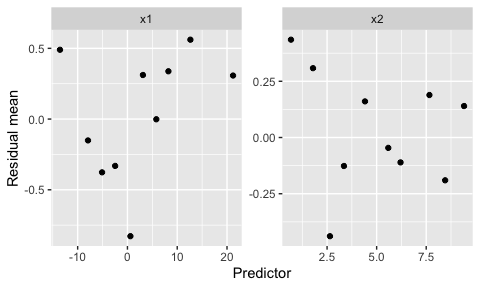This is comparable to the marginal model plots above: where the marginal model plots show a smoothed curve of fitted values and a smoothed curve of actual values, the binned residuals show the average residuals, which are actual values minus fitted values. We can think of the binned residual plot as showing the difference between the lines in the marginal model plot.

We can also bin by the fitted values of the model:

binned_residuals(fit, predictor = .fitted) |>
ggplot(aes(x = predictor_mean, y = resid_mean)) +
geom_point() +
labs(x = "Fitted values", y = "Residual mean")